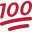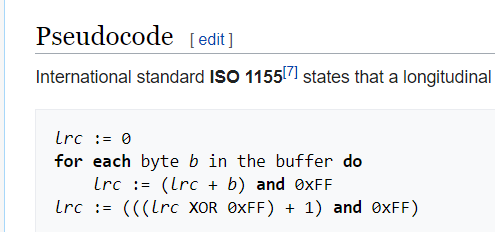# Android QuestionLCR calculate

#### Lello1964

##### Well-Known Member
Longtime User
I have to calculate LRC of a character string using this method:

LRC byte is calculated with an operation of Exclusive OR (XOR) on Data

Example :
uint8 LRC(const uint8* str, const uint16 length)
{

uint16 i=length;
uint8 r=0x00;
while (i--)
r^=*str++;
return r;
}

can you help me ?

Longtime User

#### emexes

##### Expert
I have to calculate LRC of a character string using this method:
can you help me ?

I can try.B4X:
``````'''uint8 LRC(const uint8* str, const uint16 length)
Sub LRC(A() As Byte, L As Int) As Byte

'''uint8 r=0x00;
Dim R As Byte = 0

'''uint16 i=length;
'''while (i--) {
For I = 0 to L - 1
'''r^=*str++;
R = Bit.Xor(R, A(I))
Next

'''return r;
Return R

End Sub``````

and your first major confusion will be that B4A Bytes are Java Bytes are signed (-128 to 127) rather than unsigned (0 to 255)

and the second tidy-up is that you can use A.Length to get the side of A() ie no need for the second parameter

and what's kinda funny is that the B4A compiler is going to convert our code straight back to a curly-braces language

#### Star-Dust

##### Expert
Longtime User
perhaps it is better to start from the Java code that is present on Wikipedia rather than from the other code precisely to avoid this difference in types

Last edited:
••Lello1964 and DonManfred

#### Lello1964

##### Well-Known Member
Longtime User
B4X:
``````#if JAVA
Public byte calculateLRC(byte[] data) {
byte checksum = 0;
For (int i = 0; i <= data.length - 1; i++) {
checksum = (byte) ((checksum + data[i]) & 0xFF);
}
checksum = (byte) (((checksum ^ 0xFF) + 1) & 0xFF);
Return checksum;
}
#End If``````

I tried this java code but it gives me compile error:

src\b4a\example11\main.java:437: error: <identifier> expected
Public byte calculateLRC(byte[] data) {
^
1 erro

#### emexes

##### Expert
Probably better to get it working in regular B4A first, rather than adding complexity and confusion eg:

C:
``````Public byte calculateLRC(byte[] data) {
byte checksum = 0;
For (int i = 0; i <= data.length - 1; i++) {
checksum = (byte) ((checksum + data[i]) & 0xFF);
}
checksum = (byte) (((checksum ^ 0xFF) + 1) & 0xFF);
Return checksum;
}``````

producing a different check-value than:

Example :
uint8 LRC(const uint8* str, const uint16 length)
{

uint16 i=length;
uint8 r=0x00;
while (i--)
r^=*str++;
return r;
}

•Lello1964

#### emexes

##### Expert
and your first major confusion will be that B4A Bytes are Java Bytes are signed (-128 to 127) rather than unsigned (0 to 255)

This is not an actual problem, just something that confuses many people when they first encounter it.

The actual bit-pattern is correct.

If the negative shades of its numeric value irk you sufficiently, then: `Log(Bit.And(SignedByte, 0xFF))`

#### Serge Bertet

##### Active Member
This post seems to be close to want you want to acheive:

#### Star-Dust

##### Expert
Longtime User
Probably better to get it working in regular B4A first, rather than adding complexity and confusion eg:

producing a different check-value than:
What I meant was to translate into B4X starting from Java and thus there will be no type compatibility problems.

The algorithm is described on Wikipedia, in the version that was posted (I don't know if it's swift or MatLab or something else) it seems that it lacks to add the value 1 at the end of the calculation.Last edited:

#### DonManfred

##### Expert
Longtime User
can you help me ?
What is the value of the bytearray (source) and what is the expected output?

The Library is based on the JAVA-Code from Wikipedia (see link in #2)

B4X:
``````    Dim lrc As LRC
Dim values() As Byte = Array As Byte(15,63,255,128)
Dim lrccrc As Byte = lrc.calculateLRC(values)
Log(\$"\$8{lrccrc}"\$)``````

#### Attachments

• LRC.zip
1.3 KB · Views: 25
Last edited:
••Lello1964 and Star-Dust

Android Question Calc CheckSum
Replies
1
Views
686
Android Question checksum ccitt
Replies
2
Views
736
Android Question UDP Checksum
Replies
26
Views
4K
Android Question BLE2 TI Sensor Tag CC2650
Replies
7
Views
2K